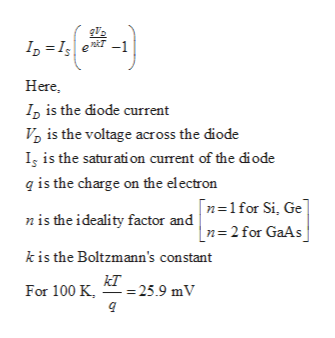# For a Silicon diode, if the value of the forward current is 10 mA and the reverse saturation current is 25 nA, then what will be the voltage across the diode at 300 K temperature?

Question
6 views

For a Silicon diode, if the value of the forward current is 10 mA and the reverse saturation current is 25 nA, then what will be the voltage across the diode at 300 K temperature?

check_circle

Step 1

Write the expression for ...help_outlineImage TranscriptioncloseI, =I e -1 Here, I, is the diode current V, is the voltage across the diode Iş is the saturation current of the diode q is the charge on the electron |n=1for Si, Ge n= 2 for GaAs nis the ideality factor and k is the Boltzmann's constant kT - = 25.9 mV For 100 K, fullscreen

### Want to see the full answer?

See Solution

#### Want to see this answer and more?

Solutions are written by subject experts who are available 24/7. Questions are typically answered within 1 hour.*

See Solution
*Response times may vary by subject and question.
Tagged in

### Electrical Engineering